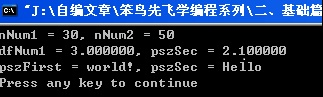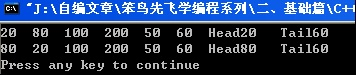# 聚星亭

posts - 74, comments - 166, trackbacks - 0, articles - 0
C++博客 :: 首页 :: 新随笔 :: 联系 :: 聚合:: 管理

C++ 提供了“模板”这一特性， 可以将“类型” 参数化， 使得编写的代码更具有通用性。 因此大家都称模板编程为 “通用编程”或 “泛型编程”。

##### 一、 函数模板

1、 函数模板的定义和使用

template <模板参数列表>

{

// code ...

}

// 定义一个函数模板， 用来实现任意类型数据的相互交换。

template <typename T> // 声明一个 数据类型， 此类型随 调用方 类型的变化而变化

void swap(T& a, T& b)

{

T tmp = a;

a = b;

b = tmp;

}

int main(int argc, char* argv[])

{

int nNum1 = 50;

int nNum2 = 30;

double dfNum1 = 2.1;

double dfNum2 = 3.0;

char *pszFirst = "Hello ";

char *pszSec = "world!";

swap <int> (nNum1, nNum2); // swap函数模板实例化为 int类型的模板函数再调用

printf("nNum1 = %d, nNum2 = %d\r\n", nNum1, nNum2);

swap<double> (dfNum1, dfNum2);

printf("dfNum1 = %f, pszSec = %f\r\n", dfNum1, dfNum2);

swap<char *> (pszFirst, pszSec);

printf("pszFirst = %s, pszSec = %s\r\n", pszFirst, pszSec);

return 0;

}Address Publics by Value Rva+Base Lib:Object 0001:00000140 ?swap@@YAXAAH0@Z 00401140 f i Exp01.obj 0001:00000190 ?swap@@YAXAAN0@Z 00401190 f i Exp01.obj 0001:000001f0 ?swap@@YAXAAPAD0@Z 004011f0 f i Exp01.obj

a、 函数模板 并不是函数，它仅在编译时根据调用此模板函数时传递的实参类型来生成具体的函数体！若 函数模板没有被调用责不生成任何代码也不对模板代码作任何语法检查。

b、 在模板类型参数中， 实参与形参要求严格匹配，它不会进行任何的类型转换。

c、 对于函数模板，我们在调用时不一定必须先进行将它实例化为一个函数也是可以的，编译器会自动的识别模板的类型。（换句话说：Exp01中的代码可以直接使用swap， 而不需要<>

2、  函数模板的重载

template <typename T>

T constMax(T const& a, T const& b)

{

return a < b ? b : a;

}

A、 当我们需要传入两个指针类型的实参时，该模板就失效了，需要重载该模板：

template <typename T>

T constMax(T* const& a, T* const& b)

{

return *a < *b ? *b : *a;

}

B、 倘若我们再需要比较两个字符串大小时，上面两个模板都失效了。因为char* 并没有提供 operator < 运行，我们只能通过调用strcmp库函数自己实现一个Max模板的特例（见Exp02）：

const charMax(const char*& a, const char*& b)

{

return strcmp(a, b) < 0 ? b : a;

}

C++模板机制规定，如果一个调用，即匹配普通函数，又能匹配模板函数的话，则优先匹配普通函数。因此，当我们模板特例化的时候，会先匹配特例化的函数。

##### 二、 类模板

1、  基本概念

struct Node

{

Nodeint nData = 0 )

{

m_nData = nData;

m_pPrev = m_pNext = NULL;

}

int   m_nData; // 数据元素

Node* m_pPrev; // 指向上一个元素的指针

Node* m_pNext; // 指向下一个元素的指针

};

class CDList

{

private:

int  m_nCount;

Node* m_pTail;

int    m_nMessage;

public:

CDList();

virtual ~CDList();

public:

int GetLen() const

{

m_nCount;

}

{

}

NodeGetTail() const

{

return m_pTail;

}

public:

bool Change(int nIndex1,int nIndex2);

void Release();

//增加

Nodeoperator[](int nIndex);

//删除

bool DeleteNodeint nIndex );

void PrintAll();

//查找

NodeFindNodeint nIndex );

};

template <typename T>

struct Node

{

Node( T Data )

{

m_Data  = Data;

m_pPrev = m_pNext = NULL;

}

T m_Data;  /通用类型的数据元素

Node<T>* m_pPrev; // 指向上一个元素的指针

Node<T>* m_pNext; // 指向下一个元素的指针

};

template <typename T>

class CDList

{

private:

int     m_nMessage; // 消息号

int     m_nCount; // 链表中 元素的数量

Node<T>* m_pTail; // 链表尾指针

public:

CDList();

virtual ~CDList();

public:

int GetLen() const

{

m_nCount;

}

{

}

Node<T>* GetTail() const

{

return m_pTail;

}

public:

bool Change(int nIndex1,int nIndex2);

void Release();

//增加

Node<T>* operator[](int nIndex);

//删除

bool DeleteNode( int nIndex );

void PrintAll();

//查找

Node<T>* FindNode( int nIndex );

};

2、  类模板的定义

Template <typename T>

Class 类名

{

// code，可以使用模板参数T来指定通用的数据类型。

}

int main(int argc, char* argv[])

{

CDList<int> MyList; // CDList实例化为一个int类型，也就是说链表中数据元素为整型

//(20) (80) 100 200 50 60

MyList.PrintAll();

MyList.Change(0,1);

MyList.PrintAll();

return 0;

}a、 类模板 同样也不是类，它仅在编译时根据实例化本模板时传递的实参来生成具体的类代码！若 类模板没有被实例化也没有被调用，那编译器不会为本模板生成任何代码也不对模板代码作任何语法检查。

3、  类模板的特化

template <>

class CDList<CStudent>

{

private:

int     m_nMessage; // 消息号

int     m_nCount; // 链表中 元素的数量

Node<CStudent>* m_pTail; // 链表尾指针

public:

bool Change(int nIndex1,int nIndex2);

void Release();

//增加

Node<CStudent>* operator[](int nIndex);

//删除

bool DeleteNodeint nIndex );

void PrintAll();

//查找

Node<CStudent>FindNodeint nIndex );

};

{

Node<CStudent>* pNewNode = new Node<CStudent>(Data);

if ( m_pTail )

m_pTail->m_pNext = pNewNode;

pNewNode->m_pPrev = m_pTail;

if ( m_pTail == NULL )

m_pTail = pNewNode;

m_nCount++;

return pNewNode;

}

Template<>

{

……

}

##### 三、 模板程序的组织

1、  包含方式

A、 如果模板的成员函数写在类外，则需要写成如下样式（见Exp04）：

template <typename T> // 每个类外成员函数前都要有这句

{

Node<T>* pNewNode = new Node<T>(Data);

if ( m_pTail )

m_pTail->m_pNext = pNewNode;

pNewNode->m_pPrev = m_pTail;

if ( m_pTail == NULL )

m_pTail = pNewNode;

m_nCount++;

return pNewNode;

}

B、 对于特化的代码则需要在.h文件中声明并在.cpp文件中定义，如果都写在.h文件中编译会报重定义错误。

2、  分离方式

// .h 头文件中

export template <typename T>

class CDList

{

public:

CDList();

virtual ~CDList();

public:

bool Change(int nIndex1,int nIndex2);

void Release();

//增加

Node<T>* operator[](int nIndex);

//删除

bool DeleteNode( int nIndex );

void PrintAll();

//查找

Node<T>* FindNode( int nIndex );

};

export template <typename T> // 每个类外成员函数前都要有这句

{

Node<T>* pNewNode = new Node<T>(Data);

if ( m_pTail )

m_pTail->m_pNext = pNewNode;

pNewNode->m_pPrev = m_pTail;

if ( m_pTail == NULL )

m_pTail = pNewNode;

m_nCount++;

return pNewNode;

}

.

### Feedback

@陈梓瀚(vczh)

@陈梓瀚(vczh)

1.2例子的返回值搞错了

template <typename T>
T* const& Max(T* const& a, T* const& b)
{
return *a < *b ? *b : *a;
}

@空明流转

@yuegui2

Windows编程基础是本实用的好书